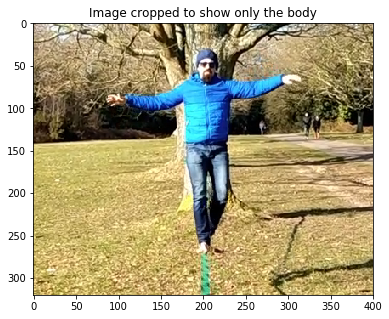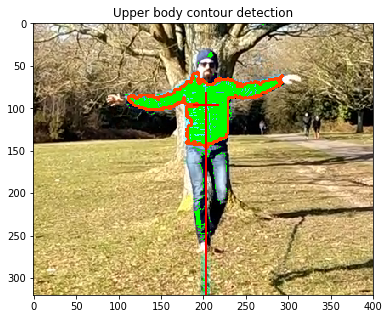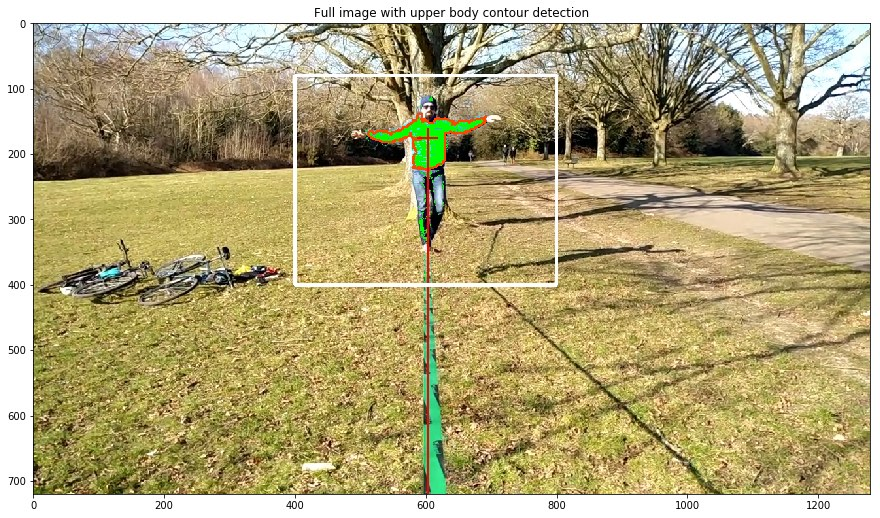OpenCV is an open source computer vision library which can be interfaced from Python. It provides lots of functionalities and is very powerful.

In this project we are interested in studying the center of mass of a person standing on a slackline. The key point is that the center of mass anybody on a slackline stays vertically on top of the contact point of the foot with the line itself, even when limbs are waving around. This can be tested empirically by recording someone walking on the line and employing OpenCV to detect body contours and find the geometric centroid of the person’s body.

As a rough example, I recorded myself walking on the line:

YouTubeVideo('fL3lAojlZY8', width=700, height=400)


Ideally, you want to wear clothes with different colours from the background, as it makes contour detection much easier. In the example video above, I achieved this partially. The blue coat is generally easy to detect, however, some shadows make it black in some areas and some detail is lost. Similarly with the jeans, they look quite black and white, hence their detection needs more care. Until I get the chance to record a better video, we will focus on detecting the upper body only, which usually provides a decent approximation.

### Import necessary libraries

%matplotlib inline
import cv2
import imutils
import numpy as np
import time
import matplotlib.pyplot as plt
from matplotlib import rcParams
rcParams['figure.figsize'] = [15, 10]


### Test contour selection

First, we will try the contour detection on a still frame from the example video. Many sophisticated contour detection techniques exist, which are outside of the scope of this short example. We will focus on colour detection, where we specify a range of RGB colours to look for, and an area of the image to do so (computationally cheaper and quicker).

In our case, we want the colour range to contain most blues, and little else. For the image area, we will broadly select the range of pixels that contain the body.

testFile = './pick_colours/test1.png'
boundaries = [([75, 0, 0], [255, 255, 70])] # OpenCV uses BGR, not RGB!
sizes = [80, 400, 400, 800]                 # In pixels

scale = 1
image = cv2.resize(image, (0, 0), fx=1./scale, fy=1./scale)
height, width, channels = image.shape
imageCropped = image[int(sizes/scale):int(sizes/scale),
int(sizes/scale):int(sizes/scale)]

plt.figure(figsize=(10,5))
plt.imshow(cv2.cvtColor(imageCropped, cv2.COLOR_BGR2RGB))
plt.title("Image cropped to show only the body")
plt.show()lower = np.array(boundaries, dtype = "uint8")
upper = np.array(boundaries, dtype = "uint8")

# Crop the image, process only the cropped bit
image[int(sizes/scale):int(sizes/scale),
int(sizes/scale):int(sizes/scale)] = imageCropped

# Find blue contours (coat)
cnts = cnts if imutils.is_cv2() else cnts
for c in cnts:
# Retain only large enough contours, this deletes outliers
if cv2.contourArea(c) > 3000:
c[:,0,0] += int(sizes/scale)
c[:,0,1] += int(sizes/scale)
# Compute the center of the contour
M = cv2.moments(c)
cX = int(M["m10"] / M["m00"])
cY = int(M["m01"] / M["m00"])
# Draw the contour and center of the shape on the image
cv2.drawContours(image, [c], -1, (0, 69, 255), 2)
cv2.line(image, (cX-15, cY), (cX+15, cY), (0, 0, 255), 2)
cv2.line(image, (cX, cY-15), (cX, height), (0, 0, 255), 2)

imageCropped = image[int(sizes/scale):int(sizes/scale),
int(sizes/scale):int(sizes/scale)]

plt.figure(figsize=(10,5))
plt.imshow(cv2.cvtColor(imageCropped, cv2.COLOR_BGR2RGB))
plt.title("Upper body contour detection")
plt.show()As we can see in the previous image, we can easily obtain a rough upper body contour. With a better choice of contour detection algorithm (maybe motion detection) and/or brigther clothes (red or orange would be ideal in this example video), a more accurate contour of the body could be obtained.

We can also find the geometric centroid of the contour, which roughly corresponds to its centre of mass (assuming uniform body density, which seems to be a good approximation). We are now ready to embed the cropped image in the full image.

# Frame the cropped image with a white rectangle
cv2.rectangle(image, (int(sizes/scale), int(sizes/scale)),
(int(sizes/scale), int(sizes/scale)),
(255, 255, 255), 3)
plt.imshow(cv2.cvtColor(image, cv2.COLOR_BGR2RGB))
plt.title("Full image with upper body contour detection")
plt.show()### Video analysis

So far, we are able to approximately find the centre of gravity in a picture of someone standing on a slackline. This can be easily extended to deal with a video instead.

The key point is that a video is just a sequence of stills (frames), so we can grab each frame of the video, apply the processing described above, and put the resulting image as a frame in an output video.

# Open video
videoInFile = './video_tests/clip1.mp4'
videoIn = cv2.VideoCapture(videoInFile)

# Find width and height
w = videoIn.get(cv2.CAP_PROP_FRAME_WIDTH);
h = videoIn.get(cv2.CAP_PROP_FRAME_HEIGHT);

# The video can be scaled down to reduce computing time
scale = 1
fps = 30
minContourSize = 3000
capSize = (int(w/scale), int(int(h/scale)/2))

# Output video
fourcc = cv2.VideoWriter_fourcc('m', 'p', '4', 'v')
videoOut = cv2.VideoWriter('output.mp4', fourcc, fps, (int(w),int(h)))

# Define the list of boundaries: blue (coat)
boundaries = [([75, 0, 0], [255, 255, 70])]
# Window frames for each colour
sizes = [[80, 400, 400, 800]]
# Centroid coordinates and masses
centroids = np.zeros([len(boundaries), 2], dtype=int)
masses = np.zeros(len(boundaries))

# Iterate over the frames of the video, one by one
while videoIn.isOpened():
image = cv2.resize(image, (0, 0), fx=1./scale, fy=1./scale)
imageTemp = image.copy()
height, width, channels = image.shape

# Loop over different colours (in our case just one, the blue coat)
for j in range(len(boundaries)):
imagePart = image[int(sizes[j]/scale):int(sizes[j]/scale),
int(sizes[j]/scale):int(sizes[j]/scale)]
lower = np.array(boundaries[j], dtype = "uint8")
upper = np.array(boundaries[j], dtype = "uint8")
imageTemp[int(sizes[j]/scale):int(sizes[j]/scale),
int(sizes[j]/scale):int(sizes[j]/scale)] = imagePart
# Find contours
cnts = cv2.findContours(mask, cv2.RETR_EXTERNAL, cv2.CHAIN_APPROX_SIMPLE) # _NONE
cnts = cnts if imutils.is_cv2() else cnts
for c in cnts:
if cv2.contourArea(c) > minContourSize/scale:
c[:,0,0] += int(sizes[j]/scale)
c[:,0,1] += int(sizes[j]/scale)
# Compute the center of the contour
M = cv2.moments(c)
cX = int(M["m10"] / M["m00"])
cY = int(M["m01"] / M["m00"])
centroids[j] = np.array([cX, cY])
masses[j] = cv2.contourArea(c)
# Draw the contour and center of the shape on the image
cv2.drawContours(imageTemp, [c], -1, (0, 69, 255), 2)

for j in range(len(boundaries)):
# Add window frames to the image to check
cv2.rectangle(imageTemp, (int(sizes[j]/scale), int(sizes[j]/scale)),
(int(sizes[j]/scale), int(sizes[j]/scale)),
(255, 255, 255), 1)
# Draw centroid lines
cv2.line(imageTemp, (centroids[j]-15, centroids[j]), (centroids[j]+15, centroids[j]), (255, 255, 255), 1)
cv2.line(imageTemp, (centroids[j], centroids[j]-15), (centroids[j], height), (255, 255, 255), 1)

# Compute mean centroid using contour centroids and their respective masses
mult = masses/sum(masses)
centroidTotal = sum((centroids.T * mult).T, 0)

try:
cv2.line(imageTemp, (int(centroidTotal)-15, int(centroidTotal)), (int(centroidTotal)+15, int(centroidTotal)), (0, 0, 255), 2)
cv2.line(imageTemp, (int(centroidTotal), int(centroidTotal)-15), (int(centroidTotal), height), (0, 0, 255), 2)
except:
pass
# Write frame to videoOut
videoOut.write(imageTemp)

# Clean up
cv2.destroyAllWindows()
videoIn.release()
videoOut.release()


Depending on the length and resolution of the video, this analysis can take a while. When the code finishes, we should have our output video file ready. Mine looks like this:

YouTubeVideo('2epc_1mojQs', width=700, height=400)


We can see that our approximated centre of mass roughly stays centered on top of the line. Of course, contour detection is not great in this example, as shadows are not captured in the coat. Also, the legs play a significant role in balance, and were not captured in our example. As mentioned earlier, with a bit of care, one could use motion detection, or combine black and white contours to pick up the legs and include them in the analysis. Orange leggings would also do the trick!The code snippets accompanying this project are available under an MIT license.